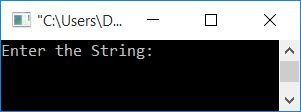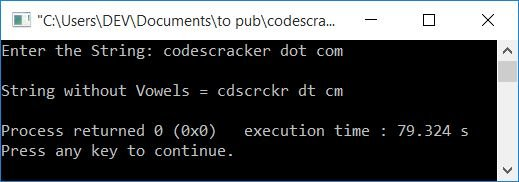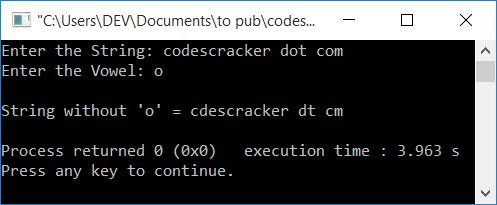# C++ Program to Delete Vowels from a String

In this article, you will learn and get code to remove (delete) vowels from strings in C++. The program is created in the following ways:

### What is a vowel?

If the pronunciation of a character is produced by humans, the breath flows out of the mouth without being blocked by teeth, lips, or tongue. Then the character is called a vowel.

There are basically 5 vowels, which are:

• a, e, i, o, and u
• A, E, I, O, and U

Sometimes, y can also be called a vowel. However, in the following program, we will only cover the vowels listed above. I'm not going into depth about vowels. We have to create the program only because we are here.

## Remove all vowels from the string

To delete all vowels from a given string in C++ programming, you have to ask the user to enter the string first. Then, as shown in the program below, search for vowels and remove them by shifting all the characters (from here) to one index back.

The question is: write a program in C++ that removes all vowels from a given string. Here is its answer:

```#include<iostream>
#include<stdio.h>
using namespace std;
int main()
{
char str;
int i=0, j, chk;
cout<<"Enter the String: ";
gets(str);
while(str[i]!='\0')
{
chk=0;
if(str[i]=='a'||str[i]=='e'||str[i]=='i'||str[i]=='o'||str[i]=='u'||
str[i]=='A'||str[i]=='E'||str[i]=='I'||str[i]=='O'||str[i]=='U')
{
j=i;
while(str[j-1]!='\0')
{
str[j] = str[j+1];
j++;
}
chk = 1;
}
if(chk==0)
i++;
}
cout<<"\nString without Vowels = "<<str;
cout<<endl;
return 0;
}```

This program was built and runs under the Code::Blocks IDE. Here is its sample run:Now supply the string "codescracker dot com" and press ENTER to remove all the vowels from it. This prints the new string without any vowels as shown in the output given below:The dry run of the above program with user input codescracker dot com goes like this:

• Initial value, i=0
• When the user enters the string, then it gets stored in the str variable in this way:
• str=c
• str=o
• str=d
• and so on, u pto
• str=m
• Because the maximum size of str is 50 but the size of the entered string is 20, a null-terminated character (\0) automatically gets assigned after the last character's index. Therefore str=\0
• Now that the while loop's condition is str[i]!='\0', str!='\0', or c!='\0', which evaluates to be true, program flow goes inside the loop.
• There, 0 gets initialized to chk. So chk=0
• And the condition (of if), str[i]=='a'||str[i]=='e'||str[i]=='i'||str[i]=='o'||str[i]=='u'|| str[i]=='A'||str[i]=='E'||str[i]=='I'||str[i]=='O'||str[i]=='U' gets evaluated.
• Because the character at str is c, which is not equal to any of the 5 vowels, the condition evaluates to be false. Therefore, program flow does not go inside the loop.
• Now, because the value of chk is 0, the value of i gets incremented. So i=1
• Now program flow goes back and evaluates the condition of the while loop again.
• This process continues until the condition is evaluated as false.
• When a character at any index of str gets matched with any of the 5 vowels, then all upcoming characters from the index where a character gets matched with a vowel get shifted to one index back.
• In this way, all the vowels gets deleted from the string.
• So after exiting from the loop, just print the value of str.

## In C++, remove only the desired vowel from a string

This program does not remove all the vowels from a string; rather, it removes only the desired one. In other words, the program prompts the user to enter the string and a vowel in order to remove the given vowel from the given string.

```#include<iostream>
#include<stdio.h>
using namespace std;
int main()
{
char str, vowel;
int i=0, j, chk;
cout<<"Enter the String: ";
gets(str);
cout<<"Enter the Vowel: ";
cin>>vowel;
while(str[i]!='\0')
{
chk=0;
if(str[i]==vowel)
{
j=i;
while(str[j-1]!='\0')
{
str[j] = str[j+1];
j++;
}
chk = 1;
}
if(chk==0)
i++;
}
cout<<"\nString without '"<<vowel<<"' = "<<str;
cout<<endl;
return 0;
}```

Here is its sample run with user input, codescracker dot com as a string, and o as the vowel to remove:#### The same program in different languages

C++ Quiz

« Previous Program Next Program »